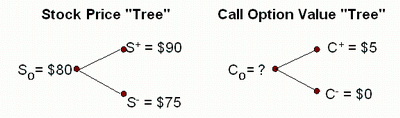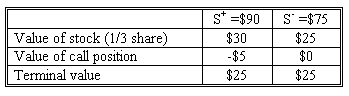### Why should I choose AnalystNotes?

AnalystNotes specializes in helping candidates pass. Period.

##### Subject 11. Binomial Valuation of Options
In finance, the binomial options model provides a generalisable numerical method for the valuation of options. The model differs from other option pricing models in that it uses a "discrete-time" model of the varying price over time of financial instruments; the model is thus able to handle a variety of conditions for which other models cannot be applied. Essentially, option valuation here is via application of the risk neutrality assumption over the life of the option, as the price of the underlying instrument evolves.

The binomial pricing model uses a "discrete-time framework" to trace the evolution of the option's key underlying variable via a binomial lattice (tree), for a given number of time steps between valuation date and option expiration. Each node in the lattice represents a possible price of the underlying at a particular point in time. This price evolution forms the basis for the option valuation. The valuation process is iterative, starting at each final node, and then working backwards through the tree to the first node (valuation date), where the calculated result is the value of the option.

Option valuation using this method is, as described, a three step process:

• price tree generation
• calculation of option value at each final node
• progressive calculation of option value at each earlier node; the value at the first node is the value of the option

We start off by having one binomial period for a European call option.

Define:

• r = risk-free rate
• c+ = Max (0, S+ - X) (call price if the stock price goes up: "up state")
• c- = Max (0, S- - X) (call price if the stock price goes down: "down-state")
• u = (S+ / S0) ("up state" price relative)
• d = (S- / S0) ("down-state" price relative)
• ST = Stock price at time (T)
• π = Greek small letter pi.

Formulas:

• π = (1 + r - d) / (u - d) (risk-neutral "up" probability)
• c0 = [π c+ + (1 - π) c-] / (1 + r) (the price of the call option)
• n = (c+ - c-) / (S+ - S-) (the hedge ratio: the number of shares of stock per option to hedge)

We assume that the stock price will only take two possible values at the expiration date of the option. In our example:

• Current stock price = S0 = \$80
• Stock price at expiration = \$90 (S+) or \$75 (S-)
• Exercise price of call option = \$85 (X)
• Time to expiration = T = 1/2 year (6 months)
• Risk-free rate of return = r = 6% (discrete and annual)

Step 1: Diagram Stock Price Dynamics and Option Values on Trees

Based on this information, tree diagrams for the stock value and call option payoffs (state dependent) would be drawn as follows:Step 2: Compute Risk Neutral Probabilities of Up and Down States

μ = (90/80) = 1.125
d = (75/80) = 0.9375
π = [(1.06)0.5 - 0.9375] / (1.125 - 0.9375) = 0.4912

Step 3: Compute Expected Value of Call Option

c0 = [0.4912 \$5 + (1 - 0.4912) \$0] / (1.06)0.5 = \$2.385

Therefore, today's value of the 1-period option is \$2.385.

Alternatively, we can use combination of Stocks and Calls to create state-independent payoffs and then determine the no-arbitrage value of the option rights.

Step 1: Calculate the hedge ratio (shares per call)

n = (\$5 - \$0) / (\$90 - \$75) = 0.3333

Step 2: Use the hedge ratio to construct a portfolio of stocks and calls in which terminal payoff is state-independent. Let TV denote the terminal value of the portfolio at expiration.

• If stock price = S-, TV- = n S- - c-
• If stock price = S+, TV+ = n S+ - c+
• TV- = TV+

Regardless of which way the underlying moves, the portfolio value should be the same (perfectly hedged).

Continuing from the previous example:

To form a perfectly hedged portfolio, an investor needs to buy 1/3 of a share of stock for each call that is written (sold), or buy 1 share of stock and sell (write) 3 calls. To see that this is true, consider the position of the portfolio at the expiration of the call if the investor writes 1 call.Note that each call is worth \$5 to the buyer or holder, so the seller of the call is in a negative position.

Step 3: Value the Option

Guaranteed outcome is \$25 for this portfolio, regardless of the value of the stock at expiration. How much should you pay for this risk-free position?

The present value of the guaranteed \$25 to be received in six months is: \$25 / 1.060.5 = \$24.28. To guarantee the \$25 outcome, the investor would have to buy 1/3 share of the stock and sell 1 call option.
\$24.28 = n S0 - c0 = initial investment ⇒ c0 = \$80/3 - \$24.28 = \$2.38

This is the same value we ended up with using the direct approach.

Suppose the option of the previous example is selling for \$3 - a clear case of price not equaling value. Investors would exploit this opportunity by selling the option and buying the underlying. The number of units of the underlying purchased for each option sold would be the hedge ratio: n = (c+ - c-) / (S+ - S-) = 0.3333. Suppose we sell 300 calls and buy 100 shares. The initial outlay would be 100 x \$80 - 300 x \$3 = \$7100. Six months later, the portfolio value will be:

• 100 x \$75 - 0 = \$7500, if stock price = \$75.
• 100 x \$90 - 300 x \$5 = \$7500, if stock price = \$90. Note that the 300 here is the number of options bought, not the hedge ratio.

Our six-month return is 7500/7100 - 1 = 5.63%, and the annualized return is (1.0563)2 - 1 = 11.58%. This risk-free return is much higher than the actual risk-free return of 6%.

If the option sells for less than \$2.38, an investor would buy the option and sell short the underlying, which would generate cash upfront. At expiration, the investor would have to pay back an amount less than 7%. All investors would perform this transaction, generating a demand for the option that would push its price back to \$2.38.

Therefore, when the option is trading at the price given by the model, a hedge portfolio would earn the risk-free rate.

If the option is a put, please note the following differences:

• Hedge ratio n = (p- - p+) / (S+ - S-)
• A risk-free hedge has the same positions in the two instruments (underlying and the put).

Learning Outcome Statements

n. explain how the value of an option is determined using a one-period binomial model;

CFA® 2022 Level I Curriculum, , Volume 5, Reading 46

User Comment
merc5559 Derivs is brutal
somehow @merc5559: wait until level 2.
Inaganti6 i wonder if i can get to level 2 !
merc5559 On Derivs level 2 now and somehow can see my comment from level 1 on this LOS. Somehow is right. Level 2 Derivatives is even worse.
thebkr777 ^Haha Yeah these are fun... Same section as level 1?
MariaDunet Formula of risk neutral probability comes from assumption that there is no arbitrage and in any case price will be U*p+D*(1-p)=1+r =>
(U-D)*p+D=1+r =>
p=(1+r-D)/(U-D)
The further D or U from 1+r, the less probability would be, eg for 1+r=15 probability of 10 is less than 13.
Allen88 Formula of risk neutral probability comes from assumption that there is no arbitrage and in any case price will be U*p+D*(1-p)=1+r =>
(U-D)*p+D=1+r =>
p=(1+r-D)/(U-D)
The further D or U from 1+r, the less probability would be, eg for 1+r=15 probability of 10 is less than 13.
jperez049 Thanks Allen88